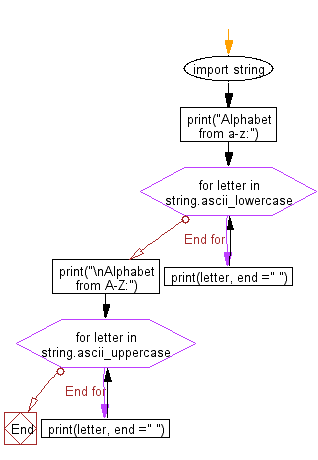﻿ Python: Print letters from the English alphabet from a-z and A-Z - w3resource# Python: Print letters from the English alphabet from a-z and A-Z

## Python Basic - 1: Exercise-114 with Solution

Write a Python program to print letters from the English alphabet from a-z and A-Z.

Sample Solution:

Python Code:

``````import string
print("Alphabet from a-z:")
for letter in string.ascii_lowercase:
print(letter, end =" ")
print("\nAlphabet from A-Z:")
for letter in string.ascii_uppercase:
print(letter, end =" ")
``````

Sample Output:

```Alphabet from a-z:
a b c d e f g h i j k l m n o p q r s t u v w x y z
Alphabet from A-Z:
A B C D E F G H I J K L M N O P Q R S T U V W X Y Z
```

Pictorial Presentation:Flowchart:Python Code Editor:

Have another way to solve this solution? Contribute your code (and comments) through Disqus.

What is the difficulty level of this exercise?

Test your Programming skills with w3resource's quiz.

﻿

## Python: Tips of the Day

What is the difference between Python's list methods append and extend?

append: Appends object at the end.

```x = [1, 2, 3]
x.append([4, 5])
print (x)
```

Output:

```[1, 2, 3, [4, 5]]
```

extend: Extends list by appending elements from the iterable.

```x = [1, 2, 3]
x.extend([4, 5])
print (x)
```

Output:

```[1, 2, 3, 4, 5]
```

Ref: https://bit.ly/2AZ6ZFq